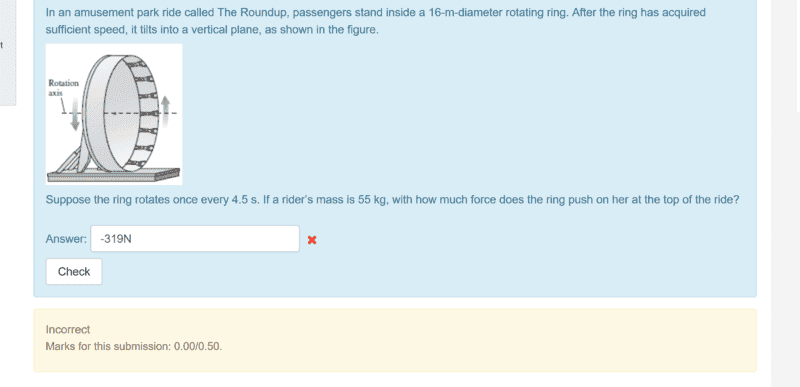# Uniform circular motion question1. Homework Statement

In picture

f=ma
v^2/r

## The Attempt at a Solution

ΣFy=-mg - Fn = m(-v^2/r)
-55*9.8 - Fn = m(-v^2/r)
v= 16π/4.5 since circumference = 2πr divide by the rotations time taken per second = 11.17m/s
-Fn = 55(-15.6) + 55*9.8
Fn = -319

What am i doing wrong here??

#### Attachments

PeterO
Homework Helper
View attachment 224234 1. Homework Statement
In picture

f=ma
v^2/r

## The Attempt at a Solution

ΣFy=-mg - Fn = m(-v^2/r)
-55*9.8 - Fn = m(-v^2/r)
v= 16π/4.5 since circumference = 2πr divide by the rotations time taken per second = 11.17m/s
-Fn = 55(-15.6) + 55*9.8
Fn = -319

What am i doing wrong here??
When the rider is in the top position, all forces are in the same direction - down.
Gravity acts down, the ring pushes down (it has to push down, since the rider is below the ring at that point) and the Nett Force is also down (towards the centre, of course, which is below/down from the rider)
Since all three forces (two acting plus one resultant) are in the same direction, it is just a simple addition of magnitudes.
Weight Force + Action Force = Resultant force
(Action Force is the force that the ring pushes with.)

BTW: when you get the answer, just say xxx.xx N down, rather than +xxx.xx N or - xxx.xxN. Otherwise you have to define what direction + or - mean.

•isukatphysics69
Ok i guess they just wanted magnitude so the answer was just 320N rounded.. now i am on the second part which is
Find the force that the ring pushes on person at the bottom of the ride.. so now the normal force is positive, mg is still negative, so
ΣFy = FN -mg = m(v^2/r)
FN =
When the rider is in the top position, all forces are in the same direction - down.
Gravity acts down, the ring pushes down (it has to push down, since the rider is below the ring at that point) and the Nett Force is also down (towards the centre, of course, which is below/down from the rider)
Since all three forces (two acting plus one resultant) are in the same direction, it is just a simple addition of magnitudes.
Weight Force + Action Force = Resultant force
(Action Force is the force that the ring pushes with.)
Thank you! got it

PeterO
Homework Helper
View attachment 224234 1. Homework Statement
In picture

f=ma
v^2/r

## The Attempt at a Solution

ΣFy=-mg - Fn = m(-v^2/r)
-55*9.8 - Fn = m(-v^2/r)
v= 16π/4.5 since circumference = 2πr divide by the rotations time taken per second = 11.17m/s
-Fn = 55(-15.6) + 55*9.8
Fn = -319

What am i doing wrong here??
I think the error is in the line
ΣFy=-mg - Fn = m(-v^2/r)
It should be
ΣFy=-mg - Fn = -m(v^2/r)

It is the Right Hand side as a whole that is negative, not just the component v. The negative sign is just a reference to direction of the quantity, not its components.

•isukatphysics69
ehild
Homework Helper
-Fn = 55(-15.6) + 55*9.8 = -319
Fn = -319

What am i doing wrong here??

-Fn = -319. What is Fn?

•isukatphysics69
-Fn = -319. What is Fn?
i see i made a stupid mistake, thank you